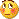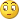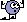# Coding help - page 321586

This is what I did in trying to apply a MA to the SSA directly, like the example in my picture.

Of course, I'm a complete noob in coding ...

So, if anybody could tell me what I have to change, I would be very grateful.

I like to solve things, even if I am a noob in this matter, but if one doesn't try to solve his problems, he will stay a noob for ever ...//+------------------------------------------------------------------+

//| FullSSA.mq4 |

//| klot@mail.ru |

//+------------------------------------------------------------------+

#import "libSSA.dll"

void fastSingular(double& a[],int n,int lag ,int s,double&b[]);

#import

#property indicator_separate_window

#property indicator_buffers 2

#property indicator_color1 White

#property indicator_color2 DeepPink

#property indicator_width1 3

#property indicator_level1 0.0

#property indicator_levelstyle STYLE_DASH

#property indicator_levelcolor Magenta

extern string note_TimeFrames ="M1;5,15,30,60H1;240H4;1440D1;10080W1;43200MN|0-CurrentTF";

extern string TimeFrame = "Current time frame";

extern int Lag = 10;

extern int NumComps = 2;

extern int PeriodNorm = 10;

extern int N = 1000;

extern int SSAMa_Mode = 3;

extern int MaPeriod = 5;

extern int Ma_Mode = 3;

extern bool Interpolate = true;

double SSA[];

double ssaWork[];

double SSAma[];

double arryTimeSeries[];

string indicatorFileName;

bool returnBars;

bool calculateValue;

int timeFrame;

int init()

{

SetIndexBuffer(0,SSA); SetIndexBuffer(1,SSAma);SetIndexStyle(0,DRAW_LINE); SetIndexDrawBegin(0,Bars-N);

indicatorFileName = WindowExpertName();

calculateValue = (TimeFrame=="calculateValue"); if(calculateValue) return(0);

returnBars = (TimeFrame=="returnBars"); if(returnBars) return(0);

timeFrame = stringToTimeFrame(TimeFrame);

IndicatorShortName(timeFrameToString(timeFrame)+ " FullSSA normalize correct");

return(0);

}

int deinit(){return(0);}

int start()

{

double dev,ma;

int nmax, nmin;

int size = N;

if (size>Bars) size = Bars;

if (ArraySize(arryTimeSeries) != size)

{

ArrayResize(arryTimeSeries,size);

ArrayResize(ssaWork,size);

}

int limit, i;

int counted_bars=IndicatorCounted();

if(counted_bars<0) return(-1);

if(counted_bars>0) counted_bars--;

limit=MathMin(Bars-counted_bars,Bars-1);

if (returnBars) { SSA = limit+1; return(0); }

if (calculateValue || timeFrame==Period())

{

for( i=size-1; i>=0; i--)

{

ma=iMA(NULL,0,PeriodNorm,0,MODE_SMA,PRICE_CLOSE,i);

dev=3*iStdDev(NULL,0,PeriodNorm,0,MODE_SMA,PRICE_CLOSE,i);

if(dev==0) dev=0.1;

arryTimeSeries=(Close-ma)/dev;//iDeMarker(NULL,0,PeriodNorm,i);

ObjectDelete("Sell"+Time);

}

fastSingular(arryTimeSeries,size,Lag,NumComps,ssaWork);

ArrayCopy(SSA,ssaWork);

nmax=ArrayMaximum(SSA,3,1);

nmin=ArrayMinimum(SSA,3,1);

if(nmax==2) {

ObjectCreate("Sell"+Time,OBJ_ARROW,0,Time,Open);

ObjectSet("Sell"+Time,OBJPROP_ARROWCODE,226);

}

if(nmin==2) {

}

//----

return(0);

}

limit =MathMax(limit,MathMin(Bars-1,iCustom(NULL,timeFrame,indicatorFileName,"","returnBars",0,0)*timeFrame/Period()));

for(i=limit; i>=0; i--)

for (i=limit; i>=0; i--) SSAma = iMAOnArray(SSA,Bars,MaPeriod,0,Ma_Mode,i);

{

int y = iBarShift(NULL,timeFrame,Time);

SSA =iCustom(NULL,timeFrame,indicatorFileName,"","calculateValue",Lag,NumComps,PeriodNorm,N,0,y);

if (timeFrame <= Period() ||y==iBarShift(NULL,timeFrame,Time)) continue;

if (!Interpolate) continue;

datetime time = iTime(NULL,timeFrame,y);

for(int n = 1; i+n =time; n++) continue;

double factor = 1.0 / n;

for(int x = 1; x < n; x++)

{

SSA= x*factor*SSA + (1.0-x*factor)*SSA;

}

}

return(0);

}

//+------------------------------------------------------------------+

string sTfTable[] ={"M1","M5","M15","M30","H1","H4","D1","W1","MN"};

int iTfTable[] = {1,5,15,30,60,240,1440,10080,43200};

//

//

//

//

//

int stringToTimeFrame(string tfs)

{

tfs = StringUpperCase(tfs);

for (int i=ArraySize(iTfTable)-1; i>=0; i--)

if (tfs==sTfTable || tfs==""+iTfTable)return(MathMax(iTfTable,Period()));

return(Period());

}

string timeFrameToString(int tf)

{

for (int i=ArraySize(iTfTable)-1; i>=0; i--)

if (tf==iTfTable) return(sTfTable);

return("");

}

//

//

//

//

//

string StringUpperCase(string str)

{

string s = str;

for (int length=StringLen(str)-1; length>=0; length--)

{

int tchar = StringGetChar(s, length);

if((tchar > 96 && tchar 223 && tchar < 256))

s = StringSetChar(s, length, tchar - 32);

else if(tchar > -33 && tchar < 0)

s = StringSetChar(s, length, tchar + 224);

}

return(s);

}[Deleted]
For slope change try something like this :[PHP]double current = iCustom(Symbol(),0,"HMA_Russian_Color",20,3,0,0);double previous1 = iCustom(Symbol(),0,"HMA_Russian_Color",20,3,0,1);double previous2 = iCustom(Symbol(),0,"HMA_Russian_Color",20,3,0,2);if (current>previous1 && previous1

Thank you Mladen!!, now i figure out forgot adding Extmapbuffer, number 2 to conditions.

How would be the condition for "while is Uptrend" buy or "while is Dntrend" then sell, as i allready have execution conditions for the orders, not for changes of slope?

Thank you again

Daniel[Deleted]

i need the condition to be "while is trending" not at exact change of slope, so i wrote this but have problem with down trend, ARE this conditions correct?? For uptrend seems working good... the same for downtrend i'm having problems...Hope you can help me, thanks.

if (current>previous1)

{

// uptrend

}

if (current<previous1)

{

// downtrend THIS IS NOT WORKING NOT TRIGGERING ANY ORDER SELL AT ALL...

}232515

Wulong10:
This is what I did in trying to apply a MA to the SSA directly, like the example in my picture.

Of course, I'm a complete noob in coding ...

So, if anybody could tell me what I have to change, I would be very grateful.

I like to solve things, even if I am a noob in this matter, but if one doesn't try to solve his problems, he will stay a noob for ever ...//+------------------------------------------------------------------+

//| FullSSA.mq4 |

//| klot@mail.ru |

//+------------------------------------------------------------------+

#import "libSSA.dll"

void fastSingular(double& a[],int n,int lag ,int s,double&b[]);

#import

#property indicator_separate_window

#property indicator_buffers 2

#property indicator_color1 White

#property indicator_color2 DeepPink

#property indicator_width1 3

#property indicator_level1 0.0

#property indicator_levelstyle STYLE_DASH

#property indicator_levelcolor Magenta

extern string note_TimeFrames ="M1;5,15,30,60H1;240H4;1440D1;10080W1;43200MN|0-CurrentTF";

extern string TimeFrame = "Current time frame";

extern int Lag = 10;

extern int NumComps = 2;

extern int PeriodNorm = 10;

extern int N = 1000;

extern int SSAMa_Mode = 3;

extern int MaPeriod = 5;

extern int Ma_Mode = 3;

extern bool Interpolate = true;

double SSA[];

double ssaWork[];

double SSAma[];

double arryTimeSeries[];

string indicatorFileName;

bool returnBars;

bool calculateValue;

int timeFrame;

int init()

{

SetIndexBuffer(0,SSA); SetIndexBuffer(1,SSAma);SetIndexStyle(0,DRAW_LINE); SetIndexDrawBegin(0,Bars-N);

indicatorFileName = WindowExpertName();

calculateValue = (TimeFrame=="calculateValue"); if(calculateValue) return(0);

returnBars = (TimeFrame=="returnBars"); if(returnBars) return(0);

timeFrame = stringToTimeFrame(TimeFrame);

IndicatorShortName(timeFrameToString(timeFrame)+ " FullSSA normalize correct");

return(0);

}

int deinit(){return(0);}

int start()

{

double dev,ma;

int nmax, nmin;

int size = N;

if (size>Bars) size = Bars;

if (ArraySize(arryTimeSeries) != size)

{

ArrayResize(arryTimeSeries,size);

ArrayResize(ssaWork,size);

}

int limit, i;

int counted_bars=IndicatorCounted();

if(counted_bars<0) return(-1);

if(counted_bars>0) counted_bars--;

limit=MathMin(Bars-counted_bars,Bars-1);

if (returnBars) { SSA = limit+1; return(0); }

if (calculateValue || timeFrame==Period())

{

for( i=size-1; i>=0; i--)

{

ma=iMA(NULL,0,PeriodNorm,0,MODE_SMA,PRICE_CLOSE,i);

dev=3*iStdDev(NULL,0,PeriodNorm,0,MODE_SMA,PRICE_CLOSE,i);

if(dev==0) dev=0.1;

arryTimeSeries=(Close-ma)/dev;//iDeMarker(NULL,0,PeriodNorm,i);

ObjectDelete("Sell"+Time);

}

fastSingular(arryTimeSeries,size,Lag,NumComps,ssaWork);

ArrayCopy(SSA,ssaWork);

nmax=ArrayMaximum(SSA,3,1);

nmin=ArrayMinimum(SSA,3,1);

if(nmax==2) {

ObjectCreate("Sell"+Time,OBJ_ARROW,0,Time,Open);

ObjectSet("Sell"+Time,OBJPROP_ARROWCODE,226);

}

if(nmin==2) {

}

//----

return(0);

}

limit =MathMax(limit,MathMin(Bars-1,iCustom(NULL,timeFrame,indicatorFileName,"","returnBars",0,0)*timeFrame/Period()));

for(i=limit; i>=0; i--)

for (i=limit; i>=0; i--) SSAma = iMAOnArray(SSA,Bars,MaPeriod,0,Ma_Mode,i);

{

int y = iBarShift(NULL,timeFrame,Time);

SSA =iCustom(NULL,timeFrame,indicatorFileName,"","calculateValue",Lag,NumComps,PeriodNorm,N,0,y);

if (timeFrame <= Period() ||y==iBarShift(NULL,timeFrame,Time)) continue;

if (!Interpolate) continue;

datetime time = iTime(NULL,timeFrame,y);

for(int n = 1; i+n =time; n++) continue;

double factor = 1.0 / n;

for(int x = 1; x < n; x++)

{

SSA= x*factor*SSA + (1.0-x*factor)*SSA;

}

}

return(0);

}

//+------------------------------------------------------------------+

string sTfTable[] ={"M1","M5","M15","M30","H1","H4","D1","W1","MN"};

int iTfTable[] = {1,5,15,30,60,240,1440,10080,43200};

//

//

//

//

//

int stringToTimeFrame(string tfs)

{

tfs = StringUpperCase(tfs);

for (int i=ArraySize(iTfTable)-1; i>=0; i--)

if (tfs==sTfTable || tfs==""+iTfTable)return(MathMax(iTfTable,Period()));

return(Period());

}

string timeFrameToString(int tf)

{

for (int i=ArraySize(iTfTable)-1; i>=0; i--)

if (tf==iTfTable) return(sTfTable);

return("");

}

//

//

//

//

//

string StringUpperCase(string str)

{

string s = str;

for (int length=StringLen(str)-1; length>=0; length--)

{

int tchar = StringGetChar(s, length);

if((tchar > 96 && tchar 223 && tchar < 256))

s = StringSetChar(s, length, tchar - 32);

else if(tchar > -33 && tchar < 0)

s = StringSetChar(s, length, tchar + 224);

}

return(s);

}

Wulong10

I do not see the picture586

So, when I take a MA from MT4 and apply it to the SSA (apply to first ind. data), the MA looks alright, when I open MT4, but when it runs in real time, it doesn't follow the SSA anymore.

This only happens on M1, on the M5 it runs without a problem.

The picture should explain it visually.

Thanks.

Files:
ssa.png  72 kb232515

Wulong10:

So, when I take a MA from MT4 and apply it to the SSA (apply to first ind. data), the MA looks alright, when I open MT4, but when it runs in real time, it doesn't follow the SSA anymore.

This only happens on M1, on the M5 it runs without a problem.

The picture should explain it visually.

Thanks.

Don't forget that SSA recalculates. If you do not calculate the MA of all the SSA bars that are recalculated then it will stop following the SSA586

Ok, now I understand, why it works on the M5, it's because I have set the period of the MA there to 1 and on the M1 I have set it to 2 ....That should solve the problem. I have tested it on my trading sim., it's not perfect, but much better !

With period 1 the MA should follow the SSA.

But how I can incorporate a MA directly in the code of the SSA ? And it should also recalculate all the SSA bars....or is that not possible ?232515

Wulong10:
Ok, now I understand, why it works on the M5, it's because I have set the period of the MA there to 1 and on the M1 I have set it to 2 ....That should solve the problem. I have tested it on my trading sim., it's not perfect, but much better !

With period 1 the MA should follow the SSA.

But how I can incorporate a MA directly in the code of the SSA ? And it should also recalculate all the SSA bars....or is that not possible ?

Wulong10

Can you please post your mq4 file too (copying text to mql almost always causes some problems)?232515

Never mind the last request

Here is a version with an added moving average : ssa__ma.mq4

Files:
ssa__ma.mq4  5 kb
ssa__ma.gif  75 kb586

Ok, thanks Mladen, you're the greatest !

I have no time to test it now, it's foot this evening, I will let you know the result ...when time is ripe.

Now I also can see how I should have done it, so I will be 0.5 % less noobish after I have studied it.